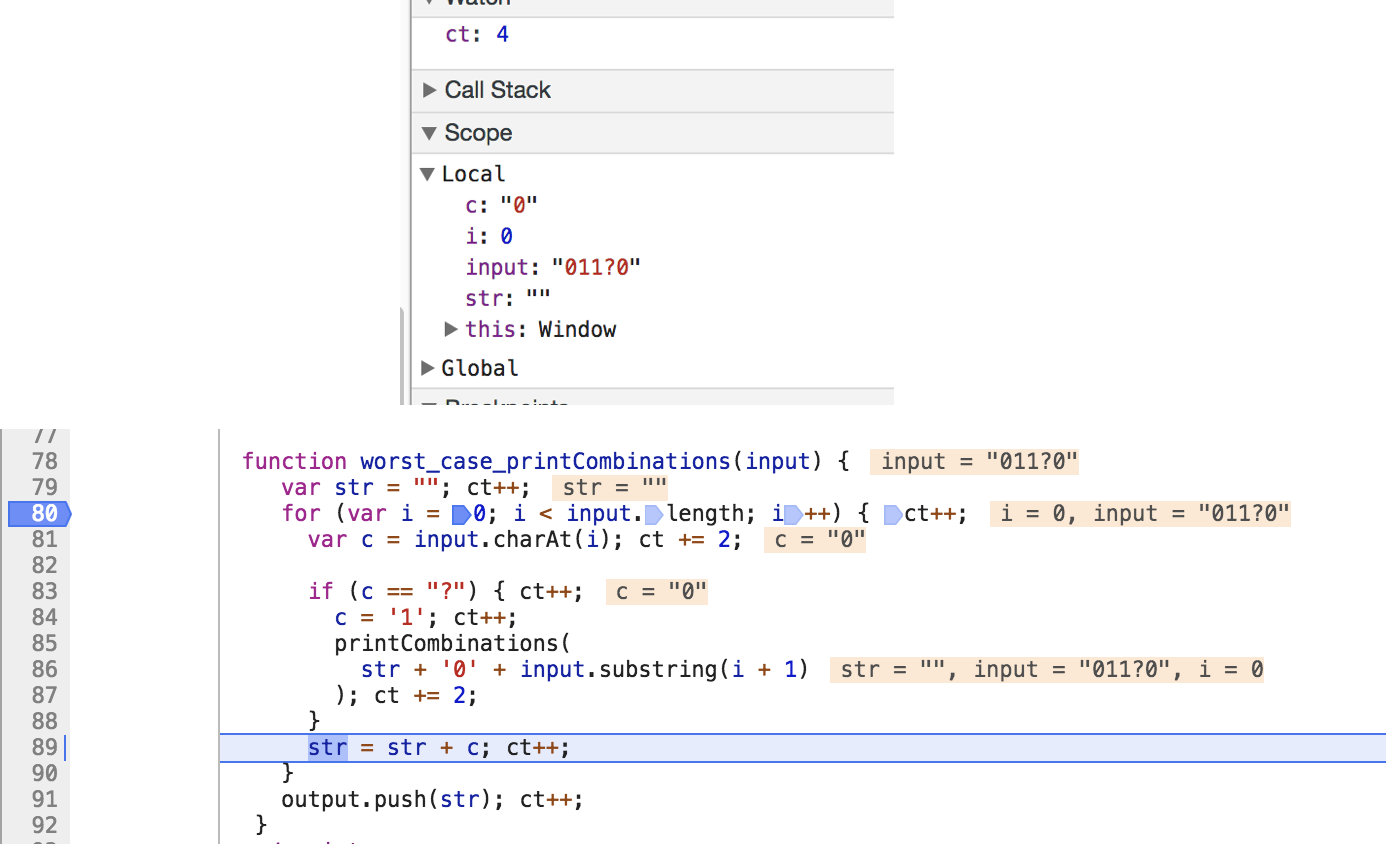`Hey 👋 new reader,Pluralsight gave me some free 1 monthsubscriptions, get them beforethey are gone!`

• interview
Part 1.

A simple technical algorithm question, asked at Google interviews taken from a user on Glassdoor.

### Question:

``````Find all string combinations consisting only of 0, 1, where ? can be either 0 or 1
``````

Input: string containing characters 0, 1 and ?, where ? is a wildcard for 0 or 1. Output: print all possible combinations of the string.

Example Input

“011?0”

Example Output

[“01100”, “01110”]

### Worst Case

To solve problems like these, a good method is to think of any solution you can think of. This is likely to be the worst case solution.

For this problem, my worst case solution is this:

Setup Code

``````var output = [];
var input = "011?0";
print(input);
console.log(output);
``````

Algorithm

``````function print(input) {
var str = "";
for(var i = 0; i < input.length; i++) {
var c = input.charAt(i);

if (c == "?") {
c = 1;
print(
str + '0' + input.substring(i + 1)
);
}
str = str + c;
}
output.push(str);
}
``````

This algorithm loops over the string and builds a new one without the ‘?’ character. When it gets to a ‘?’ character, it builds a new string using a 0 instead of this ‘?’ and continues with this run, and re-calls the algorithm with the same string but with the ‘?’ replaced with a 1.

Complexity:

`O(2^n)`, where N is the number of wildcards `?`. 2 because there are 2 options the wildcard could be.

The positives and negatives of this solution are:

Pros:

• Simple

Cons:

• Performs unnecessary calculations - every time a ? appears, the function calls itself and starts at the beginning of the string again, re-checking each character to see if it is a ‘?’.
• Uses recursion? (is this even a con?)
• Requires a global variable `output`

### Better Case

• Improved function to take in start position: `print(str, 0)`, `i` is set to start pos. When we call it again, we use: `print(str, i + 1)`
``````function print(input, output, start) {
var str = input.substring(0, start);

for (var i = start; i < input.length; i++) {
var c = input.charAt(i);

if (c == "?") {
c = 1;
print(
str + '0' + input.substring(i + 1),
output,
i
);
}
str = str + c;
}
output.push(str);
}
``````

Complexity:

`O(2^n)`, where N is the number of wildcards `?`. 2 because there are 2 options the wildcard could be.

Pros:

• Uses less computations
• Doesn’t require a global variable `output`

Cons:

• Uses recursion (is this even a con?)

### Measuring Computations

I initially thought the better case used less computations than the worst case. I decided to test this theory by adding up a counter for each line I thought needed a computation. See the source code on CodePen.

``````Input: ? - Worst Case:  Total Computations: 21
Input: ? - Better Case: Total Computations: 19 (3 less computations)

Input: ?? - Worst Case:  Total Computations: 65
Input: ?? - Better Case: Total Computations: 57 (8 less computations)

Input: ??? - Worst Case:  Total Computations: 181
Input: ??? - Better Case: Total Computations: 129 (52 less computations)

Input: ???? - Worst Case:  Total Computations: 461
Input: ???? - Better Case: Total Computations: 273 (188 less computations)
``````

Better Case counting computations

``````function print(input, output, start) {
var str = input.substring(0, start); ct += 2;

for (var i = start; i < input.length; i++) { ct += 2;
var c = input.charAt(i); ct += 2;

ct++;
if (c == "?") {
c = 1;  ct++;
print(
str + '0' + input.substring(i + 1),
output,
i
); ct += 2;
}
str = str + c;  ct++;
}
output.push(str);  ct++;
}
``````

I also discovered I had a bug in my worst case algorithm after counting these computations and debugging the function using Chrome dev tools JavaScript debugger.Chrome’s JavaScript debugger revealed my error and I fixed it.

I found two solutions to this problem, one slightly better than the other.

## Solution

``````function print(input, output, start) {
var str = input.substring(0, start);

for (var i = start; i < input.length; i++) {
var c = input.charAt(i);

if (c == "?") {
c = 1;
print(
str + '0' + input.substring(i + 1),
output,
i
);
}
str = str + c;
}
output.push(str);
}
``````